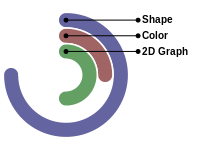# OEDrawROCSScores¶

void OEDrawROCSScores(OEDepict::OEImageBase& image,
const std::vector<double>& scores)


Renders the scores into a radial graph.

image

The image on which the radial graph of the scores is drawn.

scores

The vector of scores. The scores will expected in the following order - shape overlap score - color overlap score - 2D similarity score (optional)

Note

All scores are expected to be in the range of $$[0.0, 1.0]$$. This $$[0.0, 1.0]$$ range is transformed to $$[0.0^{\circ} - 360.0^{\circ}]$$ angles when representing the scores in the diagram.

image = oedepict.OEImage(200, 150)
scores = oechem.OEDoubleVector([0.75, 0.25, 0.50])
oegrapheme.OEDrawROCSScores(image, scores)
oedepict.OEWriteImage("DrawROCSScores.png", image)Example of using the OEDrawROCSScores function

Code Example